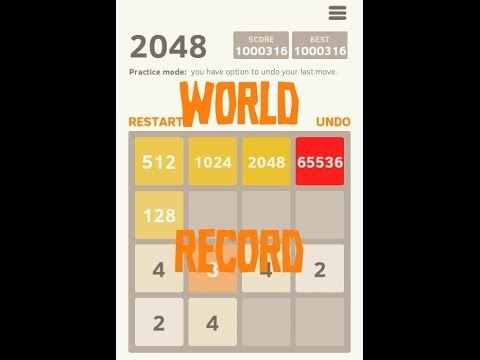2048: much harder

Algebra Level 5Assuming a perfect game, what's highest possible SCORE that can be achieved in a game of 2048 if only two's spawn.

Hint: it's not 2^16

How the game works for those who aren't familiar (although I suggest playing the game first or watching a video of it to fully understand it)

You may only combine tiles of the same number.

The grid is 4x4 and can have a maximum of 16 tiles at once.

You may not "delete" a piece from the board.

The game ends when no more tiles can be combined.

When combining two tiles of value x, the resulting tile will have a value of 2x.

Exg: if you combine two two's to make a four, then combine two more two's to create another four, then combine those two fours to get an eight, your total score will be 4+4+8=16.

When two tiles are combined, then they increase your score by the number on the new tile created.

×